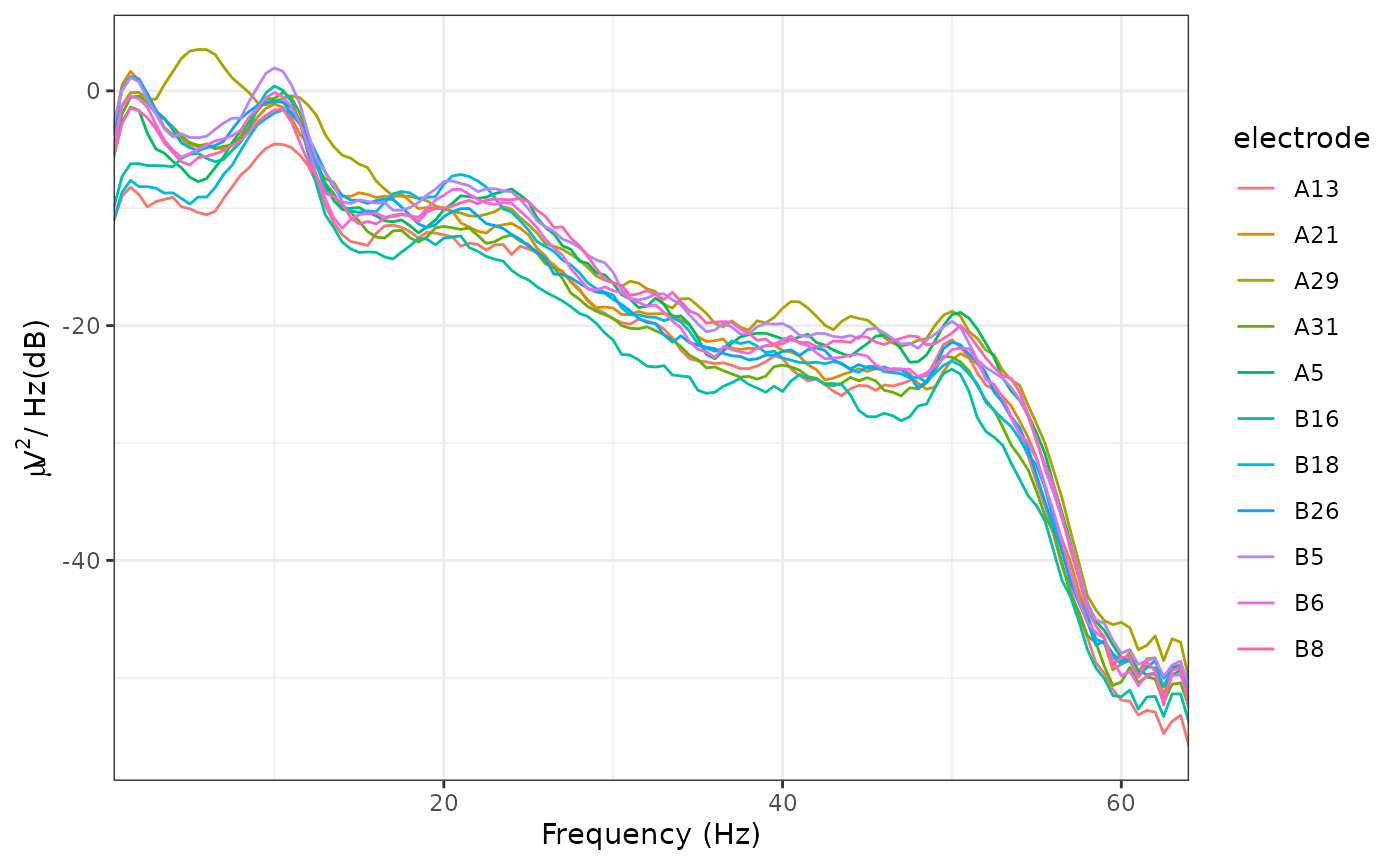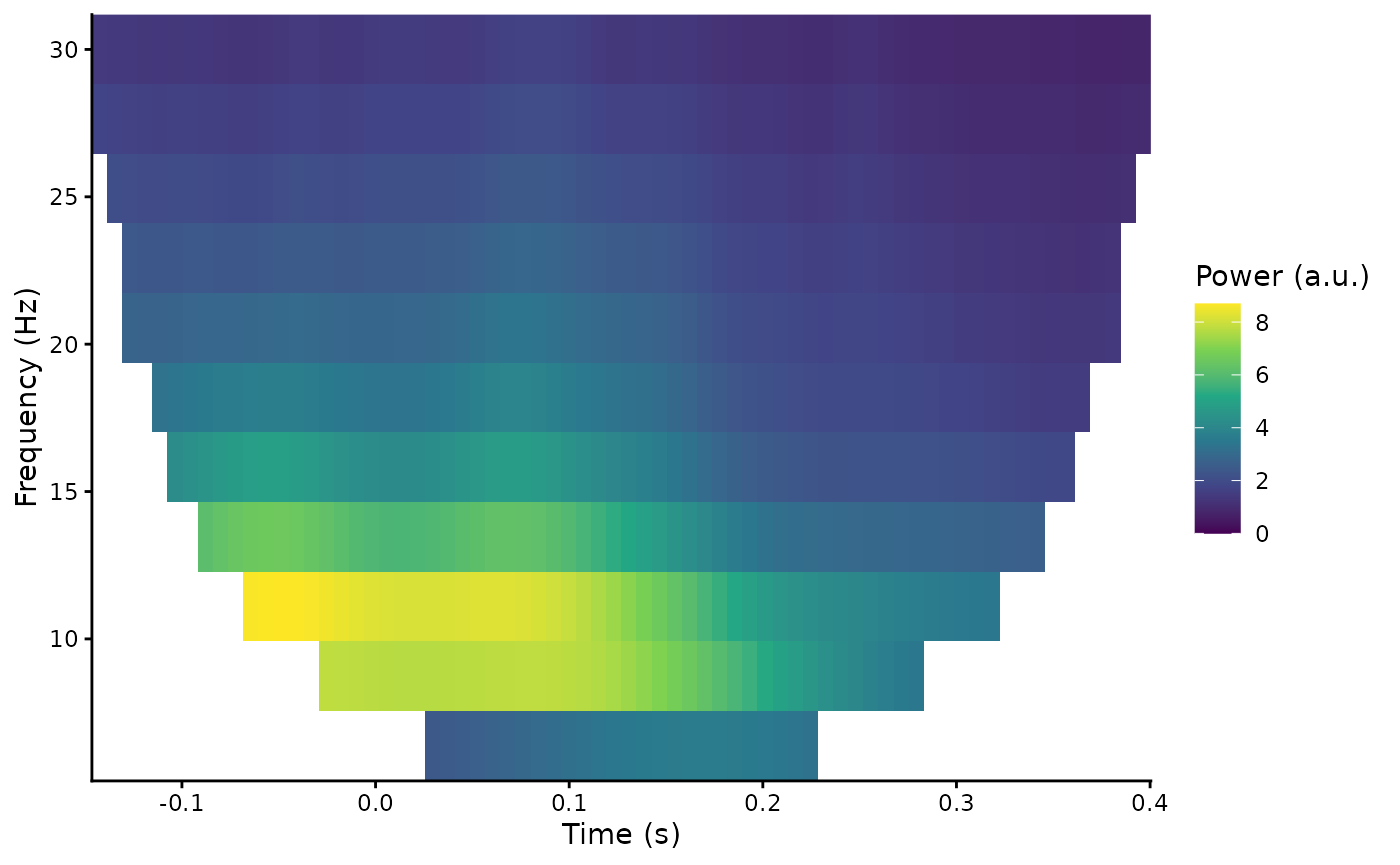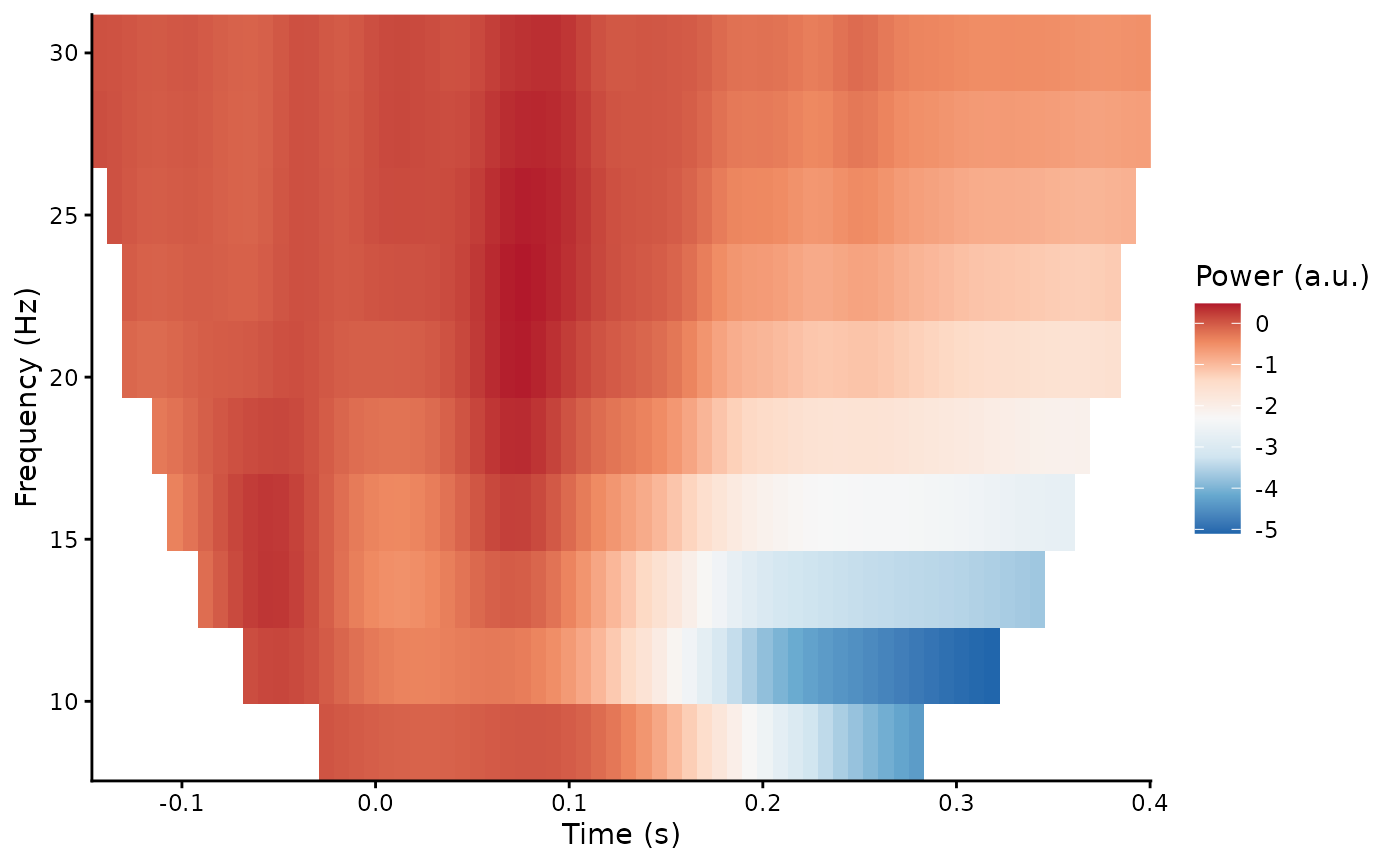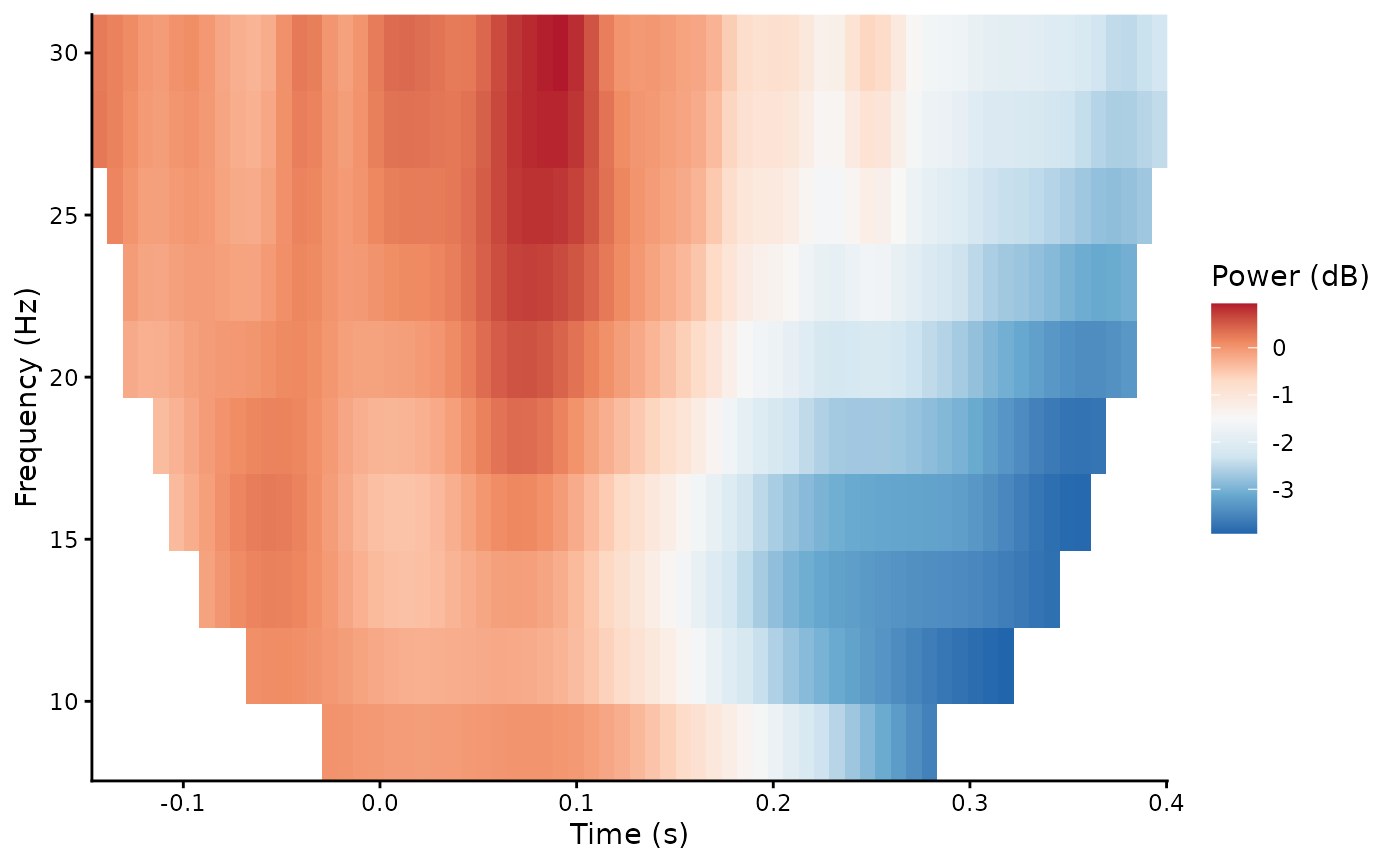Periodicity is commonly observed in EEG signals. For example, oscillations in the alpha frequency range (approximately 8-13 Hz) were one of the first signals observed in the human EEG. One method of analysing this periodicity is to calculate the Power Spectral Density using a method such as Welch’s FFT.

## Frequency analysis

In eegUtils, this can be achieved using compute_psd() and plot_psd(). With epoched data, compute_psd() calculates the PSD for each trial separately. compute_psd() returns a data.frame with spectral power at each resolved frequency and for each electrode. Note that plot_psd() can be called directly on eeg_data or eeg_epochs objects without first having to compute_psd(). With epoched data, it will average over epochs before plotting.

library(eegUtils)
#> Make sure to check for the latest development version at https://github.com/craddm/eegUtils!
#>
#> Attaching package: 'eegUtils'
#> The following object is masked from 'package:stats':
#>
#>     filter
demo_psd <- compute_psd(demo_epochs)
#> Removing channel means per epoch...
#> Joining, by = "epoch"
plot_psd(demo_epochs)
#> Removing channel means per epoch...## Time-frequency analysis

Frequency analysis necessarily discards temporal information. One problem is that it assumes stationarity - that the signal remains stable in terms of frequency and power across the whole analysed time window. However, this is rarely the case with EEG data;

Time-frequency analysis is a method of accounting for non-stationarity by decomposing the signal using a moving-window analysis, tiling the time-frequency space to resolve power over relatively shorter time-windows.

In eegUtils, compute_tfr() can be used to calculate a time-frequency representation of eeg_epochs(). Currently, this is achieved using Morlet wavelets. Morlet wavelets are used to window the signal, controlling spectral leakage and time-frequency specificity. Morlet wavelets have a user-defined temporal extent, which in turn determines the frequency extent. We define the temporal extent of our wavelets by cycles; we define it as an integer number of cycles at each frequency of interest.

demo_tfr <- compute_tfr(demo_epochs,
method = "morlet",
foi = c(4, 30),
n_freq = 12,
n_cycles = 3)
#> Output frequencies: 4 6.36 8.73 11.09 13.45 15.82 18.18 20.55 22.91 25.27 27.64 30
#> Removing channel means per epoch...
demo_tfr
#> Epoched EEG TFR data
#>
#> Frequency range      :    4 6.36 8.73 11.09 13.45 15.82 18.18 20.55 22.91 25.27 27.64 30
#> Number of channels   :    11
#> Electrode names      :    A5 A13 A21 A29 A31 B5 B6 B8 B16 B18 B26
#> Number of epochs :    None, averaged.
#> Epoch limits     :    -0.197 - 0.451 seconds
#> Sampling rate        :    128  Hz

Note that the characteristics of the wavelets, in terms of temporal and frequency standard deviations, are stored inside the object:

demo_tfr$freq_info$morlet_resolution
#>      sigma_f    sigma_t
#> 1   1.333333 0.11936621
#> 2   2.121212 0.07503019
#> 3   2.909091 0.05470951
#> 4   3.696970 0.04305011
#> 5   4.484848 0.03548725
#> 6   5.272727 0.03018456
#> 7   6.060606 0.02626057
#> 8   6.848485 0.02323944
#> 9   7.636364 0.02084172
#> 10  8.424242 0.01889249
#> 11  9.212121 0.01727669
#> 12 10.000000 0.01591549

The results of the time-frequency transformation can be plotted using the plot_tfr() function.

plot_tfr(demo_tfr)Baseline correction is common in the literature. Several different methods are possible, both for plotting only, and as a modification to the object using rm_baseline.

plot_tfr(demo_tfr, baseline_type = "absolute", baseline = c(-.1, 0))plot_tfr(demo_tfr, baseline_type = "db", baseline = c(-.1, 0))